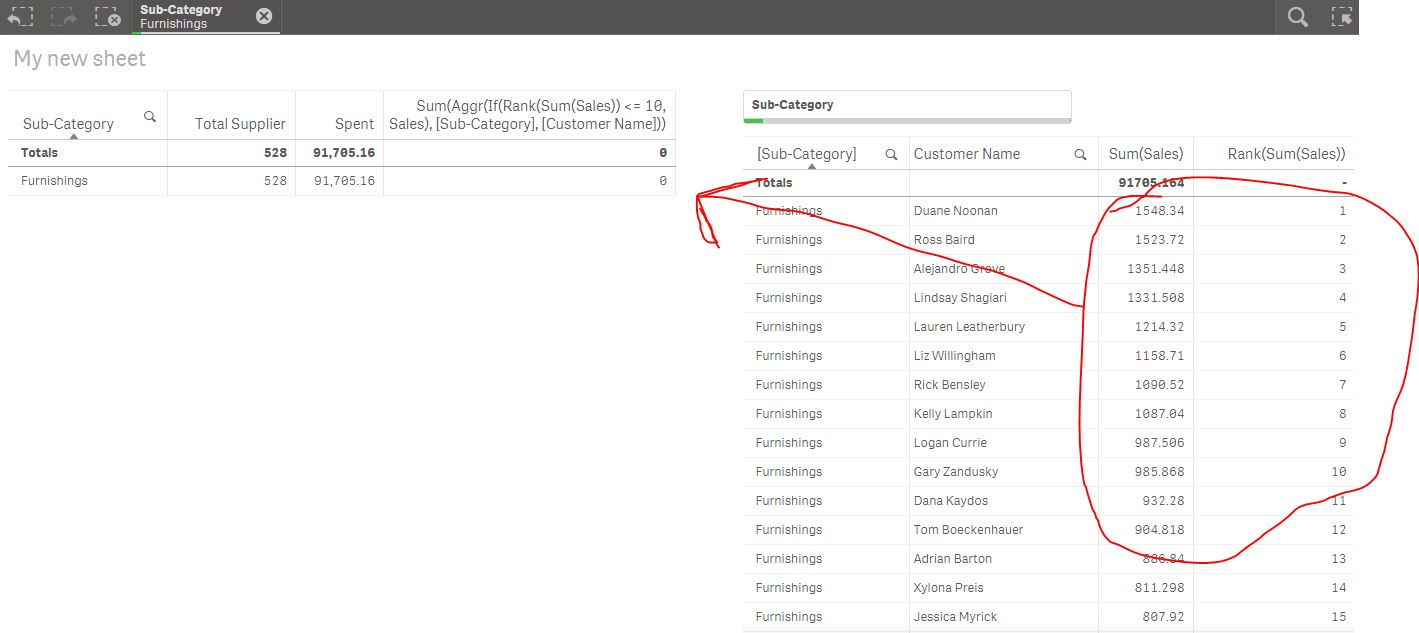New to Qlik Sense

Discussion board where members can get started with Qlik Sense.

AnnouncementsPartner

How to find sum Top n value of each dimension?

Hello Qlikers,

Greetings..!!

I wanted to find spend of top 2 customers for each category. I tried using the expression,

Sum({<CUSTOMER = {"=rank(Aggr(sum(Value),CUSTOMER,CATEGORY))<=2"}>} Value)

this expression is working fine when I select any category but when I remove selection expected value isn't correct. I have attached sample .qvf with this discussion for your convenience.

Looking for help..!!

Thanks and Regards,

Mohan

Tags (3)
8 RepliesMVP

Re: How to find sum Top n value of each dimension?

Try this

Sum(Aggr(If(Rank(Sum(Value)) <= 2, Value), CATEGORY, CUSTOMER))Partner

Re: How to find sum Top n value of each dimension?

Hi Sunny,

Thanks for your response. Your expression works good in the attached sample data, but it doesn't work in my actual application. Do you have any idea why?

Thanks,

MohanMVP

Re: How to find sum Top n value of each dimension?

It is giving you an error or what exactly is the issue?Partner

Re: How to find sum Top n value of each dimension?

Sunny, It is not giving any error. The issue is the expression isn't giving expected output in my application but it seems to be good in the sample application you have attached.MVP

Re: How to find sum Top n value of each dimension?

I guess tell us what you are getting and what you expect to see. Unless you give those details, I will have no idea what might be going on.Partner

Re: How to find sum Top n value of each dimension?

Hi sunny,

Please let me know if the snippets aren't explaining better.If you see this sheet, I used the expression you suggested. Expression output isn't giving the correct value for the category like furniture, paper, phones, etc.

I have crosschecked the same by filter the category,I hope you're clear on my issue.

I checked the format of the Sales, everything is fine.

Regards,

MohanMVP

Re: How to find sum Top n value of each dimension?

Try this may be

Sum(Aggr(If(Rank(Sum(Value)) <= 2, Sum(Value)), CATEGORY, CUSTOMER))Partner

Re: How to find sum Top n value of each dimension?

Sunny,

Thank you very much. Now, I'm getting the expected output.

Regards,

Mohan